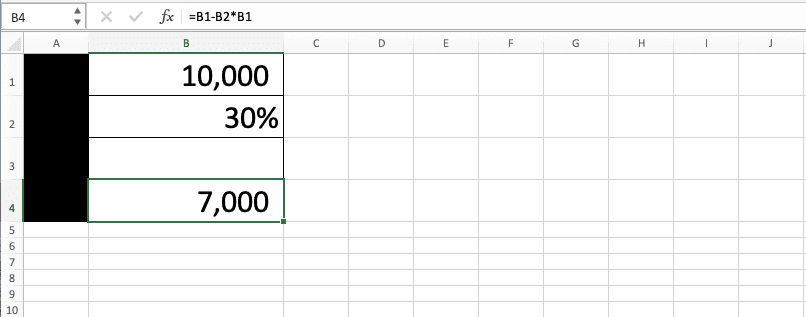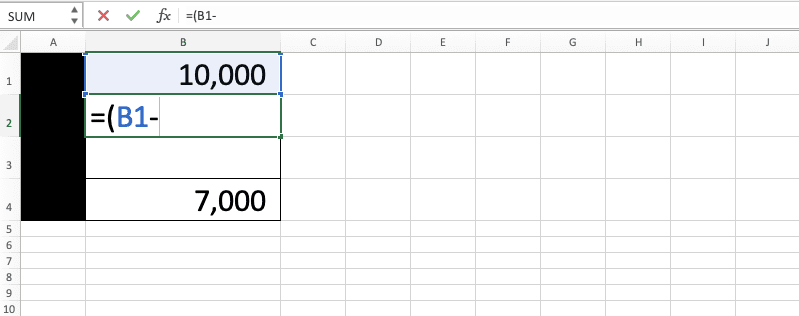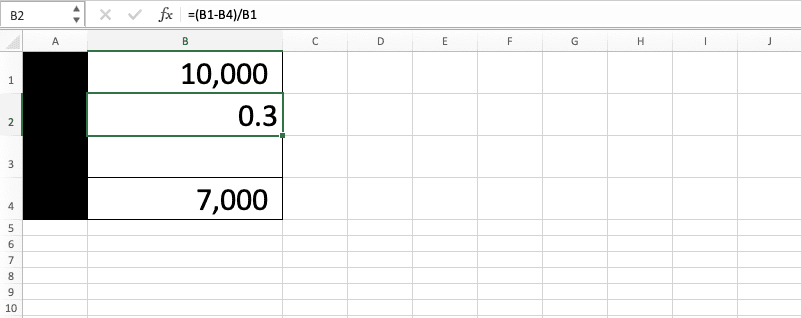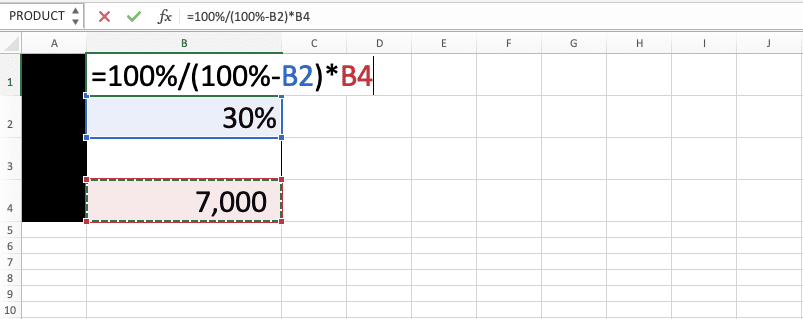How to Calculate Discount in Excel: Examples and Formulas - Compute Expert

# How to Calculate Discount in Excel: Examples and Formulas

Home >> Excel Tutorials from Compute Expert >> Excel Tips and Trick >> How to Calculate Discount in Excel: Examples and Formulas

In this tutorial, you will learn completely about how to calculate discounts in excel.

The variables usually considered in a discount calculation are the discounted price, discount percentage, and original price (before discount). Here, we will discuss how the three of them can be calculated using formula writings in excel.

Disclaimer: This post may contain affiliate links from which we earn commission from qualifying purchases/actions at no additional cost for you. Learn more

## Brief Description of Discount Calculation in Excel

Discount calculation in excel is one of the calculations often used in this software. By using excel, the calculation process related to discount can be done easier and faster.

For each of the three discount variables calculation, you must know the value of the other two first. This is because the calculation process for each of them needs the other two variables as its references.

To help you understand better about discount calculation in excel and the three variables relationship, you can see the example below. From there, we can infer how the variables are connected and can be used to get the value of one another.## The Calculation of the Discounted Price

The first variable we discuss here is the discounted price. Generally, the calculation writing to get the discounted price in excel can be written as follows.

=original_price - discount_percentage * original_price

The final writing and result example can be seen below:As seen in the general writing and example’s formula bar, you need to subtract the original price with the discount value. The discount value itself can be gotten by multiplying your discount percentage with the original price. From there, you can get the discounted price that you want.

In detail, the steps to write the calculation process of the discounted price in excel are as follows:

1. Type the equal sign ( = ) in the cell where you want to place the discounted value2. Input the original price or the cell coordinate where the number is after =. Then, type in a minus sign ( - )3. Input the discount percentage or the cell coordinate where the percentage is. Make sure the percentage symbol ( % ) is behind the input you put in here. Then, type a star sign ( * )4. Input the original price or the cell coordinate where the number is5. Press Enter
6. Done!After learning how to calculate the discounted price, next we will see how the discount percentage variable is calculated.

## The Calculation of the Discount Percentage

Generally, the writing of discount percentage calculation in excel can be given as follows:

=(original_price - discounted_price) / original_price

And here is an example of the writing usage and its result (look at the formula writing in the formula bar).From here, we can see what are the things you need to write in the discount percentage calculation. You need to get the discount value first by subtracting the discounted price from the original price. After that, compare the discount value with the original price to get your discount percentage.

The steps to calculate the discount percentage in excel can be explained in detail as follows:

1. Type the equal sign ( = ) in the cell where you want to put the discount percentage2. Type an open bracket sign then input the original price or the cell coordinate where the number is. Then, type a minus sign ( - )3. Input the discounted price or the cell coordinate where the number is. Then, type a close bracket sign4. Type a slash sign ( / ) as the symbol of division/comparison5. Input the original price or the cell coordinate where the number is6. Press Enter7. If the result isn’t a percentage yet, click Percent Style in the Home tab on the cell where the result is8. Done!After knowing how to calculate the discount percentage in excel, we will discuss how to calculate the original price last.

## The Calculation of the Original Price

Generally, the writing of the formula to calculate the original price in excel is as follows.

=100% / (100% - discount_percentage) * discounted_price

The example of the writing result in excel is as follows.From the general writing and example, we can see how the calculation of the original price is done. You need to multiply the discounted price with the 100% reverse of your discount percentage to get the original price.

Next, the writing steps will be explained in detail.

1. Type the equal sign ( = ) in the cell where you want to put in the original price2. Type 100% then type a slash sign ( / )3. Type an open bracket sign then type the 100% percentage again. Then, type a minus sign ( - )4. Input the discount percentage or cell coordinate where the number is. Make sure your input here already has a percent sign ( % ) behind it. Then, type a close bracket sign and a star sign ( * ) as the multiplication symbol5. Input the discounted price or the cell coordinate where the number is6. Press Enter
7. Done!## Exercise

After you learn the tutorials above, now let’s practice and deepen your understanding by doing the exercise below!

### Question

What are the numbers that must be filled on each row in the gray-colored cells? (the data per row is independent of each other)

To change the decimal number’s number in your result, you can use the increase decimal/decrease decimal menu in the Home tab. But, before that, don’t forget to put your cursor on the cell where your result is.Or right-click after you put the cursor and choose Format Cells. In the Number tab in the dialog box shown, choose Number. Change the value in the Decimal places according to the decimal number’s number you want before clicking OK.However, please remember that this method only changes the decimal interface of your calculation result. If you want to really change the value, then you need to use the number rounding formulas in excel. These formulas tutorials can be seen here!

Related tutorials you should learn:

Get updated excel info from Compute Expert by registering your email. It's free!